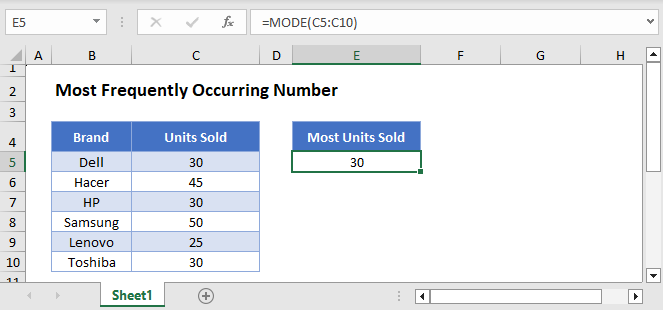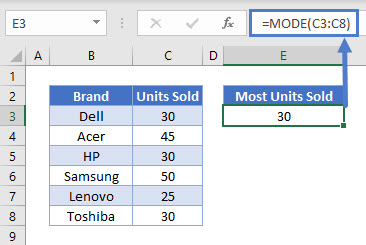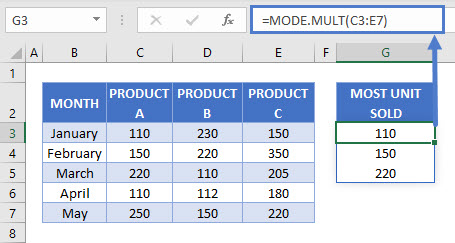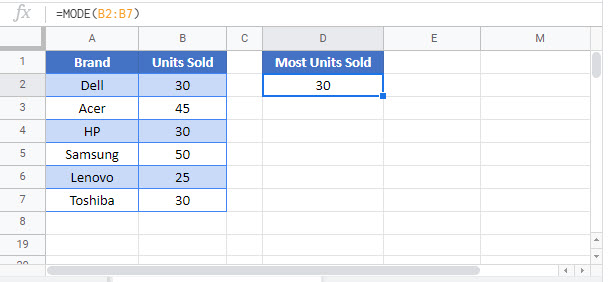# Find the Most Frequent Numbers – Excel & Google Sheets

This tutorial will demonstrate how to determine the most frequently occurring number in a range in Excel and Google Sheets.## Single Most Frequent Number

We can calculate the most frequent number in a range by using the MODE Function.

``=MODE(C3:C8)``In the example above, the MODE Function selected the number that occurred the most within the specified range, which is 30.

Note: The MODE Function has been “replaced” with the MODE.SNGL Function. However, the MODE Function is still available for backwards compatibility. The Functions work exactly the same.

## Multiple Frequent Numbers

What happens if you have more than one number that occurs the most number of times? The MODE Function will simply pick which result to show, ignoring the others. Instead, you can use the MODE.MULT Function to output all of the most frequently occurring numbers.

``=MODE.MULT(C3:E7)``The MODE.MULT Function does what its name implies, it shows the multiple numbers with the highest number of occurrences.

In the example above, the numbers 110, 150 and 220 occurred 3 times equally within the range. In using the MODE.MULT Function we did not only get the first most frequent number, but all three numbers that were the most frequent numbers within the specified range.

## Most Frequently Occurring Number in Google Sheets

These formulas work exactly the same in Google Sheets as in Excel.### Excel Practice Worksheet

Practice Excel functions and formulas with our 100% free practice worksheets!